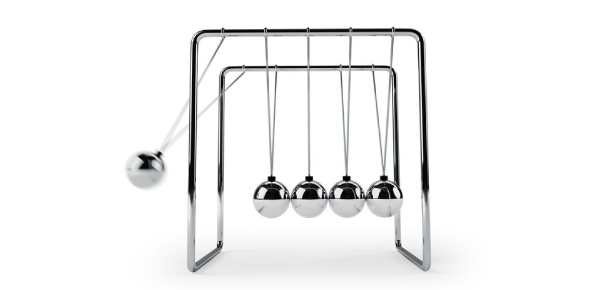# JEE Main: Laws Of Motion Set 1 Quiz! Physics

9 Questions | Attempts: 134
ShareSettings.

• 1.
A block of mass 15 kg is suspended by three strings as shown in the figure. Choose the correct option on the basis of the diagram. (Take g = 10 m/s2)
• A.

TA = 400N, TB = 500N

• B.

TA = 200N, TB = 250N

• C.

TA = 302.5N, TB = 225N

• D.

TA = 225N, TB = 112.5N

• 2.
A block of mass M is suspended on the side ‘A’ of a pulley with a string. A monkey of mass 2M is climbing on the side B with a uniform acceleration of g/4 (with respect to rope). If there is no friction between pulley and string and between string and monkey then what will be the tension in the string?
• A.

13/18 Mg

• B.

3/2 Mg

• C.

4 Mg

• D.

5/2 Mg

• 3.
A block of mass M1 is attached to a string of mass M2 and length L. If a horizontal force F is applied on the string to pull the block then what will be the tension at the midpoint of the string?
• A.
• B.
• C.
• D.
• 4.
A lift is going up and the change in the speed of the lift is shown with the help of the diagram. If the total mass of the lift with passenger is 1600 kg then what will be the tension in the rope pulling the lift at time t = 9 seconds.
• A.

17700 N

• B.

15000 N

• C.

12000 N

• D.

12300 N

• 5.
A car of mass 1500 kg collides with another car (In the state of rest) of mass 1350 kg due to which the second car starts moving with an acceleration of 4 . What will be the acceleration of the first car?
• A.

4.8 m/s2

• B.

7.75 m/s2

• C.

5.2

• D.

3.6

• 6.

• A.
• B.
• C.
• D.
• 7.
In the arrangement shown in the figure, the block of mass ‘m’ is moving upward with acceleration ‘a’. The tension in all the string is same. If whole system starts from rest then what will be the value of velocity ‘v’ after 2 seconds?
• A.
• B.
• C.
• D.
• 8.
Three forces F1, F2 and F3 are acting on a block of mass ‘M’. The box is in the state of equilibrium and force F2 is perpendicular to the force F3. Force F1 is removed and due to it, the box starts moving with some acceleration. What should be the value of acceleration?
• A.
• B.
• C.
• D.
• 9.
A pendulum, with a bob of mass ‘m’, is hung from the ceiling of a car. The car is ascending on an inclined plane with an angle of inclination = 450. If the car is moving with an acceleration a= g, then what will be the angle subtended by the string of the pendulum with the normal to the ceiling in equilibrium.
• A.
• B.
• C.
• D.

## Related TopicsBack to top
×

Wait!
Here's an interesting quiz for you.